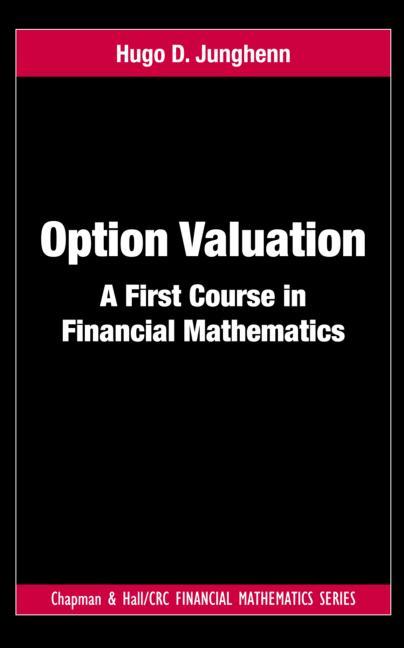Option Valuation: A First Course in Financial Mathematics

1st Edition

Hugo D. Junghenn

Chapman and Hall/CRC
Published November 23, 2011
Textbook - 266 Pages - 10 B/W Illustrations
ISBN 9781439889114 - CAT# K14090
Series: Chapman and Hall/CRC Financial Mathematics Series

For Instructors Request Inspection Copy

was \$72.95

USD\$58.36

SAVE ~\$14.59

FREE Standard Shipping!

Preview

Summary

Option Valuation: A First Course in Financial Mathematics provides a straightforward introduction to the mathematics and models used in the valuation of financial derivatives. It examines the principles of option pricing in detail via standard binomial and stochastic calculus models. Developing the requisite mathematical background as needed, the text presents an introduction to probability theory and stochastic calculus suitable for undergraduate students in mathematics, economics, and finance.

The first nine chapters of the book describe option valuation techniques in discrete time, focusing on the binomial model. The author shows how the binomial model offers a practical method for pricing options using relatively elementary mathematical tools. The binomial model also enables a clear, concrete exposition of fundamental principles of finance, such as arbitrage and hedging, without the distraction of complex mathematical constructs. The remaining chapters illustrate the theory in continuous time, with an emphasis on the more mathematically sophisticated Black-Scholes-Merton model.

Largely self-contained, this classroom-tested text offers a sound introduction to applied probability through a mathematical finance perspective. Numerous examples and exercises help students gain expertise with financial calculus methods and increase their general mathematical sophistication. The exercises range from routine applications to spreadsheet projects to the pricing of a variety of complex financial instruments. Hints and solutions to odd-numbered problems are given in an appendix and a full solutions manual is available for qualifying instructors.

Instructors

We provide complimentary e-inspection copies of primary textbooks to instructors considering our books for course adoption.